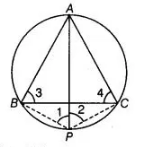# If ABC is an equilateral triangle inscribed`
Question:

If ABC is an equilateral triangle inscribed in a circle and P be any point on the minor arc BC which does not coincide with B or C, then prove that PA is angle bisector of ∠BPC.

Solution:

Given ΔABC is an equilateral triangle inscribed in a circle and P be any point on the minor arc BC which does not coincide with B or C.

To prove PA is an angle bisector of ∠BPC.

Construction Join PB and PC.Proof Since, $\triangle A B C$ is an equilateral triangle.

$\angle 3=\angle 4=60^{\circ}$

Now, $\angle 1=\angle 4=60^{\circ}$ .....(i)

[angles in the same segment $A B$ ]

$\angle 2=\angle 3=60^{\circ}$ $\ldots$ (ii)

[angles in the same segment $A C$ ]

$\therefore$ $\angle 1=\angle 2=60^{\circ}$

Hence, $P A$ is the bisector of $\angle B P C$.

Hence proved.